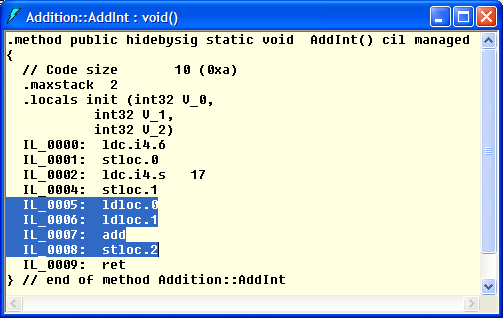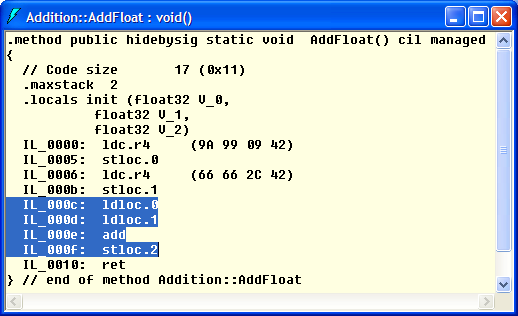# Common Intermediate Language (CIL)

### Example "untyped instructions"

While Java Bytecode instructions always specify the type of their arguments, CIL instructions often omit this.
In the example we compare the instructions for loading, adding and storing of integer and floating-point numbers in CIL and Java Bytecode.

Therefore we use a class `Addition` with two static methods that load the values of two local integer or floating-point variables, respectively, add them and then store the result in a third local variable.

 ```public class Addition { public static void AddInt () { int i1 = 6; int i2 = 17; int res = i1 + i2; } public static void AddFloat () { float f1 = 34.4f; float f2 = 43.1f; float res = f1 + f2; } public static void Main () { AddInt(); AddFloat(); } }```

With the IL disassembler (`ildasm.exe`) we can inspect the CIL code of these two methods.The Java version of class `Addition` is almost identical to the C# version.

 ```public class Addition { public static void addInt () { int i1 = 6; int i2 = 17; int res = i1 + i2; } public static void addFloat () { float f1 = 34.4f; float f2 = 43.1f; float res = f1 + f2; } public static void main (String[] args) { addInt(); addFloat(); } }```

The Java class file disassembler (`javap.exe`) shows the Java Bytecode instructions.
``````>javap Addition -c
...
Code:
0:   bipush  6
2:   istore_0
3:   bipush  17
5:   istore_1
9:   istore_2
10:  return

Here we can immediately see the different instructions for loading, adding and storing of integer (`i...`) and floating-point (`f...`) values in the Java Bytecode.